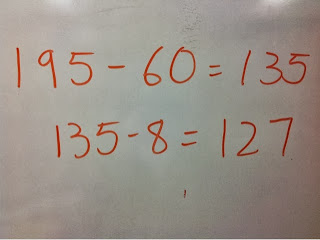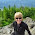## Friday, September 27, 2013

### Multi-digit Addition and Subtraction and the Common Core part 1

One of the things I am finding teachers are struggling with as we transition to the common core is how and when to teach multi-digit addition and subtraction.

Here is a look at grade 1

## Use place value understanding and properties of operations to add and subtract.

• CCSS.Math.Content.1.NBT.C.4 Add within 100, including adding a two-digit number and a one-digit number, and adding a two-digit number and a multiple of 10, using concrete models or drawings and strategies based on place value, properties of operations, and/or the relationship between addition and subtraction; relate the strategy to a written method and explain the reasoning used. Understand that in adding two-digit numbers, one adds tens and tens, ones and ones; and sometimes it is necessary to compose a ten.
• CCSS.Math.Content.1.NBT.C.5 Given a two-digit number, mentally find 10 more or 10 less than the number, without having to count; explain the reasoning used.
• CCSS.Math.Content.1.NBT.C.6 Subtract multiples of 10 in the range 10-90 from multiples of 10 in the range 10-90 (positive or zero differences), using concrete models or drawings and strategies based on place value, properties of operations, and/or the relationship between addition and subtraction; relate the strategy to a written method and explain the reasoning used.

## Use place value understanding and properties of operations to add and subtract.

• CCSS.Math.Content.2.NBT.B.5 Fluently add and subtract within 100 using strategies based on place value, properties of operations, and/or the relationship between addition and subtraction.
• CCSS.Math.Content.2.NBT.B.6 Add up to four two-digit numbers using strategies based on place value and properties of operations.
• CCSS.Math.Content.2.NBT.B.7 Add and subtract within 1000, using concrete models or drawings and strategies based on place value, properties of operations, and/or the relationship between addition and subtraction; relate the strategy to a written method. Understand that in adding or subtracting three-digit numbers, one adds or subtracts hundreds and hundreds, tens and tens, ones and ones; and sometimes it is necessary to compose or decompose tens or hundreds.
• CCSS.Math.Content.2.NBT.B.8 Mentally add 10 or 100 to a given number 100–900, and mentally subtract 10 or 100 from a given number 100–900.
• CCSS.Math.Content.2.NBT.B.9 Explain why addition and subtraction strategies work, using place value and the properties of operations.1 *explainations my be supported by drawings or objects

## Use place value understanding and properties of operations to perform multi-digit arithmetic.¹

• CCSS.Math.Content.3.NBT.A.1 Use place value understanding to round whole numbers to the nearest 10 or 100.
• CCSS.Math.Content.3.NBT.A.2 Fluently add and subtract within 1000 using strategies and algorithms based on place value, properties of operations, and/or the relationship between addition and subtraction.

## Use place value understanding and properties of operations to perform multi-digit arithmetic.

WOW!!!! There are a lot of expectations about how kids are supposed to use multi-digit computation in addition and subtraction.  Notice the STANDARD ALGORITHM is not used in the standards until GRADE 4!!!!!

So what does all this other stuff mean?

I think it means we need to help kids learn to think about math rather than just doing it.  I have shared some ideas around this on my blog before but I will be taking a closer look at this important idea and providing a lot of examples of how I am attacking this issue in grades 1-4.

Today we are going to take a peak at some strategies being used by students a few weeks into third grade.  This was done as a warm up to another lesson and took about 10 minutes.  I started kids off with a problem string culminating in the problem I wanted to discuss in further detail.  Here is what the string looked like

195 - 10 =
195 - 30 =
195 - 6 =
195 - 9 =
195 - 68 =

I pose the problems in the string one at a time and give kids a few seconds of quiet think time.  When they are ready they give me a signal and when most if not all kids are ready I ask for an answer and how they did it.  We obviously spend more time discussing different strategies as the problems increase in difficulty.  By the time we got to my culminating problem, I had kids turn and talk to a neighbor when all students were ready.  While kids are talking with a neighbor, I get a chance to listen in on different strategies.  I then know what kids I want to call on during our full group discussion.  When I call on a student, I often have them explain their partner's strategy to me.  This does wonders for student engagement and makes it so that they actually listen when their partner is talking.  As we have the whole group discussion, a student or myself will record the speaker's thinking on the board.  Here is a look at the three most common strategies used by my students.The most popular strategy and the one most apt to be suggested by the problem string  was to subtract the tens and then the ones in 2 steps.  Some kids provide further detail into their thinking and describe decomposing the 8 into 5 and 3 more and talking about how they subtracted 60 and than 5 and than 8.The students who used this strategy decomposed 195 into 190 +5.  They then put the 5 to the side for a minute and subtracted 60 to get 130.  Then they put the 5 back before subtracting the 8.  This strategy led to a great discussion about when to add a number back versus when we need to take more away.This student thought about adding up.  Because of some hard work on their part last year, these kids are fluent with combinations of 100 and were easily able to follow this students' thinking.  He started at 68 and added 32 to get up to 100 and then added 95 more to get to 195.  Then he added 95 and 32 by adding the tens and then the ones.

Be sure to follow my blog so you can see part 2!!!  Coming sometime next week:)

How would your students solve these problems?

1.